# Fixed Costs

Cost that is not dependent on changes in the production of goods and services.

Fixed costs refer to a cost that is not dependent on changes in the production of goods and services. It is also called indirect or overhead costs.With variable costs, it constitutes the total cost. Some cost accounting methods, like activity-based cost, allocate these costs to businesses for a purpose.

Overhead costs are an expense a company must pay even if no production is happening; it is not affected by changes in the goods and services produced or sold.

These costs are not directly incurred in the production process, so they are called indirect costs. Depreciation is considered a very common fixed cost that can be recorded as an indirect expense in the business's financial statements.

It can be calculated using the following formula: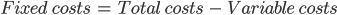Where total and variable cost is given.

It plays an integral role in the break-even analysis and cost-structure analysis. It can be calculated using the break-even analysis formula as follows: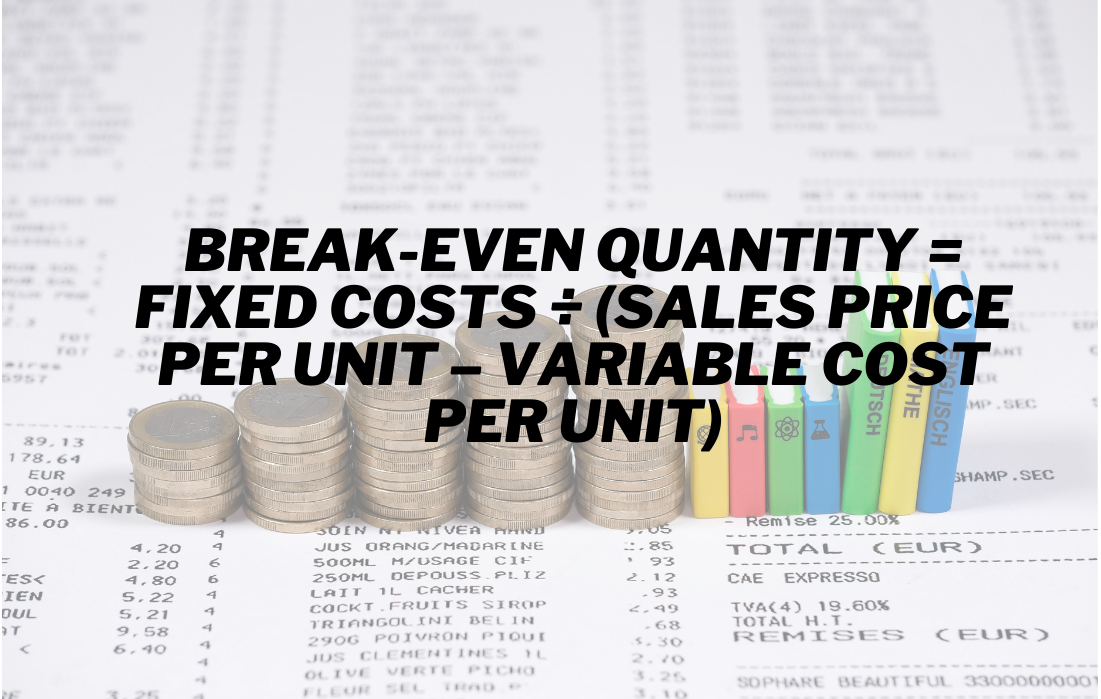Some examples of overhead costs are:

• Rent and lease costs
• Insurance payments
• Tax on property
• Loan repayments
• Salaries of full-time workers or management
• Utilities

## Understanding fixed costs (FC)

These costs are considered less controllable than variable costs, which change with the changes in the production level of goods and services. Hence, they remain constant at any point of production in the firm.

Average fixed cost (AFC) is the FC per unit measured by the total fixed cost (TFC) divided by the output level. The formula is as follows: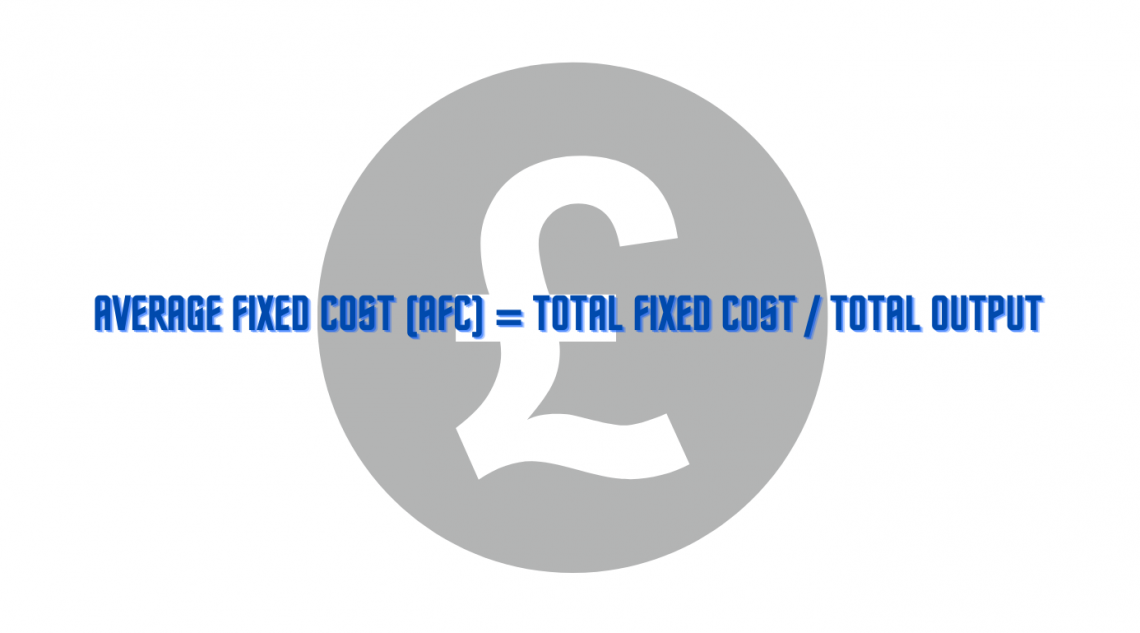These costs are affected by several factors:

2. Changes in technology applied
3. Sale of manufacturing equipment
4. Decisions to buy or sell the company assets
5. Field of services

FCs are reviewed as an independent variable allocated on the accrual basis of accounting in the business.

All sunk costs are considered fixed costs, but not all are considered sunk costs, as they cannot be recovered. These costs are also considered irrelevant in contrast to variable costs, which are considered relevant costs

If these costs rise in the long run, this situation could lead the company to exit the market and close the business, as these costs are mandatory.

Higher FC can negatively impact profits. Thus these costs can help the business make decisions regarding products and their characteristics.

The company's operating leverage is also affected by fixed and variable costs. The proportion of fixed costs to variable costs needs to be measured. Hence, higher costs facilitate a rise in operating leverage.Also, companies with higher operating leverage produce higher profits on additional units produced. The formula can calculate this: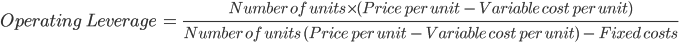1. Easier for accounting purposes
2. Remains constant irrespective of the production level
3. Enhances stability of the firm
4. Easy for auditing

1. Must be observed with care
2. Has an impact on the profitability of the firm.
3. Negative effect on profits when sales decline

## Examples

There are several examples of determining these costs and calculating the same using various formulas. Following are some of the examples which help more in understanding these costs.

Example 1:

Following are some given values,

• Total cost = \$250,000
• Variable cost per unit = \$10
• Number of units produced = 10,000
• Calculate the fixed costs for the company.

FC = Total cost - (Variable cost per unit  Number of units produced)

= \$250,000 - (\$10 X 10,000)

= \$250,000 - \$100,000

= \$150,000

Example 2:

Calculate average fixed costs (AFC) by following details:

• Total cost for producing shirts = \$150,000
• Variable costs = \$50,000
• Number of shirts produced/ total output = 1000FC = Total costs - Variable costs

= \$150,000 - \$50,000

= \$100,000

AFC = Total fixed costs / Total output

= \$100,000 / 1,000

= \$100

Example 3:

Calculation of fixed costs using break-even analysis and the following given details:

• Sales per unit = \$20
• Variable cost per unit = \$10
• TFC = \$280,000
• Total units = 1500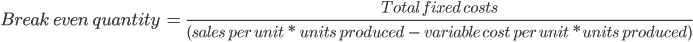= \$280,000 / (\$20 * 1500 - \$10 * 1500)

= \$280,000 / (\$30,000 - \$15,000)

= \$280,000 / \$15,000

= 18.66

Example 4:

State which one of the following is considered fixed costs:

1. Loan repayments
2. Direct labor
3. Insurance payments
4. Direct materials
5. Property tax
6. Rent costs
7. Mortgage costs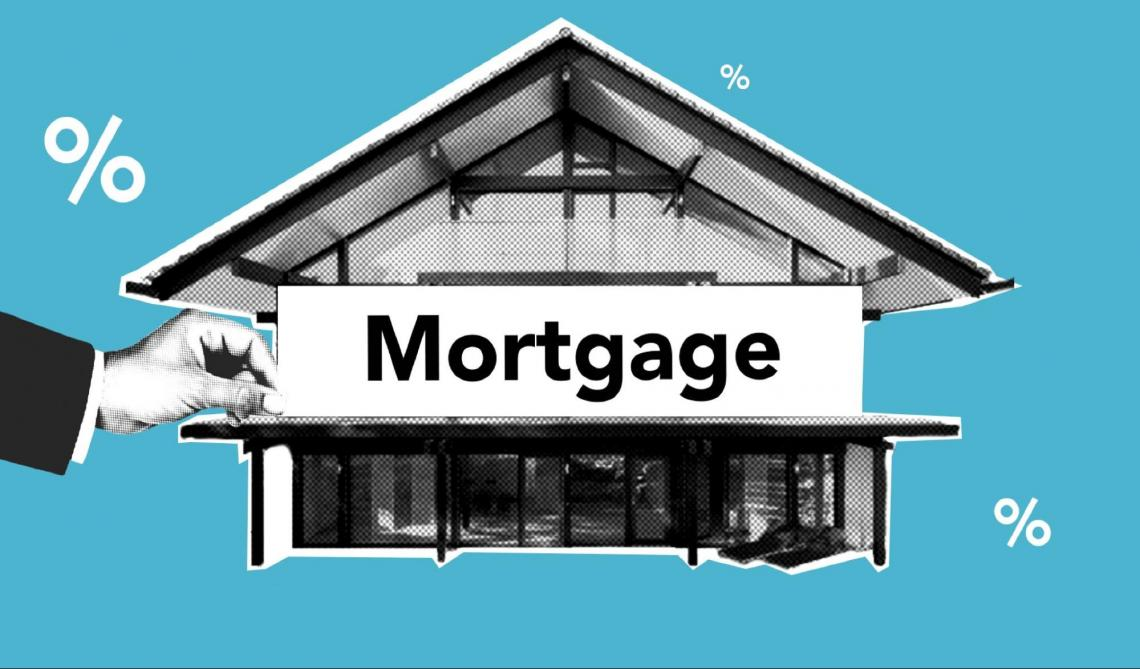The following are considered:

a) Loan repayments

c) Insurance payments

e) Property tax

f) Rent costs

g) Mortgage costs

Key Takeaways
• Fixed costs remain the same with the levels of volume of production in the firm.
• It is also known as irrelevant, overhead, and indirect costs.
• It remains constant throughout the production levels.
• It is mandatory to be paid even with zero output from production.
• It has an impact on the profitability of the company in the long run.
• Several examples include rent, mortgage, salaries, and property tax.### Everything You Need To Build Your Accounting Skills

To Help You Thrive in the Most Flexible Job in the World.

Researched and authored by Vanshika Nakul | LinkedIn

Reviewed & edited by Divya Ananth | LinkedIn